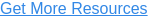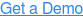# DebtBook's Premium/Discount Amortization Methodology Explained

DebtBook’s new Premium/Discount Amortization feature gives clients the ability to track their amortization of original issuance premium/discount (“OIP” or “OID”) within their DebtBook profile. Utilizing data shown in our “Maturity Detail” tab in your Issue Detail view, DebtBook programmatically calculates the original issue premium/discount on a maturity-by-maturity basis, allowing us to seamlessly generate your premium/discount amortization schedules once you select your amortization method.

Via DebtBook’s Accounting settings, users have the ability to select one of four methodologies for amortizing their premium and discount: (1) “Effective Interest Rate to Maturity”, (2) “Effective Interest Rate to Call”, (3) “Straight-Line”, or (4) “Straight-Line by Maturity”. Below we walk through how we calculate each methodology and why we support these four methodologies specifically.

## Updates to Penny Rounding (April 5, 2023)

As part of our 4/5/2023 release, DebtBook updated our rounding protocol on all Premium/Discount methodologies. The update results in immaterial penny amount rounding amounts that were previously allocated to the final amortization amount in the schedule to being allocated throughout the life of the amortization schedule.  This update provides a level of precision and predictability when amortizing premium/discount that will enable us to achieve more advanced calculations surrounding refundings and the associated impacts it has to Premium/DIscount amortizations as we move forward in calendar year 2023.

To give an example of what the update entails and the immaterial impact it will have, the below chart shows our 2022 Series amortized using the Straight-Line method under our new rounding methodology in the columns to the left, and our previous rounding methodology in the columns on the right. As we can only amortize amounts to two decimal places, a Straight-Line amount of \$849,195.75 amortized on every period would result in a total amount amortized of \$32,340,204.81, or  \$0.16 greater than our actual starting balance of \$32,340,204.65. As shown in the chart below, previously in the application we would resolve that \$0.16 discrepancy in the final period, in this case on 8/1/2041 (see blue highlighted cell below). Our enhanced methodology distributes the \$0.16 throughout the life of the amortization, generating a more consistent result regardless of which frequency and as of date you select.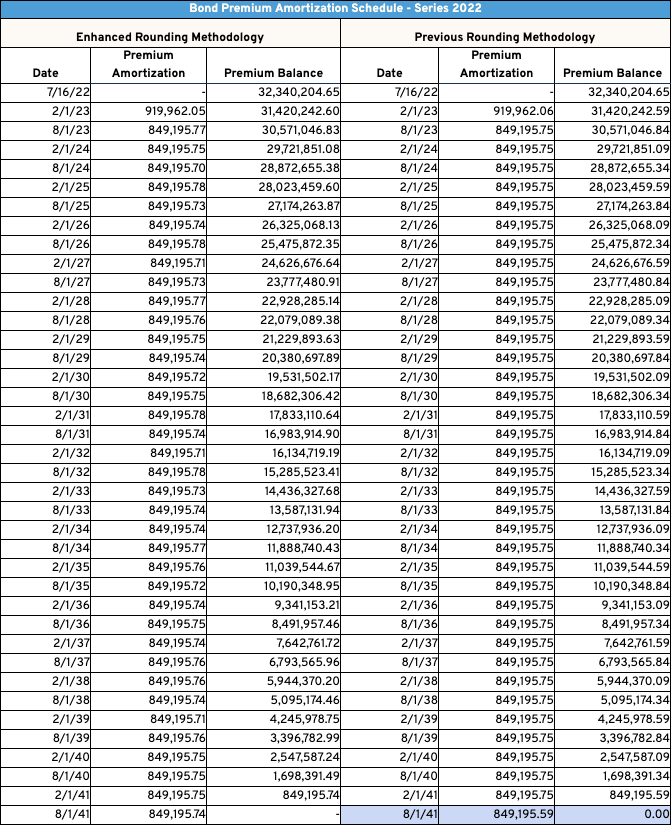## Effective Interest Rate to Maturity

Effective Interest Rate to Maturity GASB No. 62 states that any original issue discount or premium on a bond be amortized over the life of the bond utilizing the “interest method” as stated below:

“The objective of the interest method is to arrive at a periodic interest cost (including amortization) that will represent a level effective rate on the sum of the face amount of the debt and (plus or minus) the unamortized premium or discount and expense at the beginning of each period. The difference between the periodic interest cost so calculated and the nominal interest on the outstanding amount of the debt is the amount of periodic amortization.”

DebtBook’s Effective Interest Rate methodology reflects this “interest method” as referenced in GASB 62. While we know a majority of governmental issuers utilize the “Straight-Line” method due to its simplicity, DebtBook’s Premium/Discount feature will allow you to comply with the GASB standard by simply selecting the “Effective Interest Rate to Maturity” method in your Accounting Settings.

The Effective Interest Rate to Maturity method calculates a premium/discount amortization for each maturity on a stand-alone basis, then combines these values to generate a total amortization schedule for the issue in whole.

How it’s Calculated. The Effective Interest Rate method compares a bond’s Face Value Stated Interest to the bond’s Book Value Effective Interest, let’s walk through what each of these terms mean and how they are calculated using our Series information pictured below.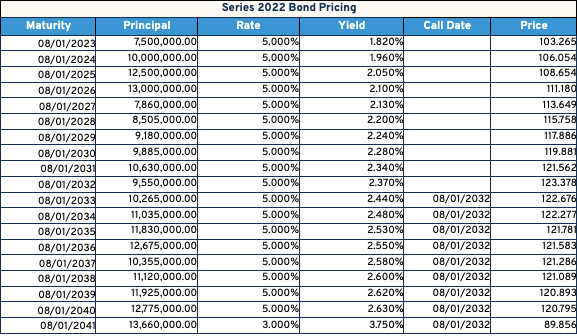Face Value Interest. Face value interest simply means the actual interest paid through the life of the maturity. Using our examples above, the stated interest is outlined in the below chart for our 8/1/2035 and 8/1/2041 maturities.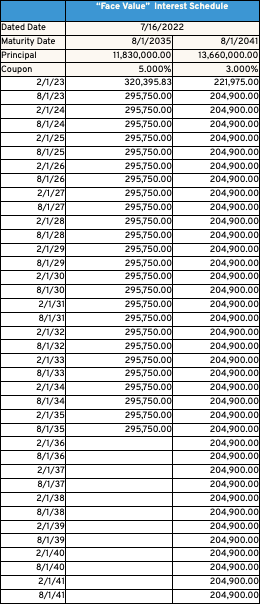Book Value Effective Interest. Book Value Effective interest is calculated similar to traditional Face Value Interest, but uses different inputs. “Book Value” represents the Par Amount of a maturity plus/(minus) the outstanding premium/discount amount on the maturity. Using our previous examples, see below for the initial book value of our bonds maturing on 8/1/2035 and 8/1/2041.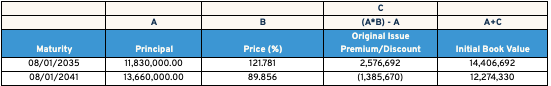As we amortize the premium/discount over the life of the bond, the book value is reduced back to its original par amount at the maturity date of the bond.

To calculate the Book Value Effective Interest, the Outstanding Book Value on a maturity is multiplied by that maturity’s Yield to Maturity. Note for callable premium bonds the Yield to Maturity is a different yield than the Stated Yield you would see in your offering document. Our 8/1/2035 maturity has a stated yield of 2.53%, which represents the yield assuming the bond is called on 8/1/2032, as compared to the Yield to Maturity which assumes the bond is outstanding until maturity. Our Yield to Maturity for our 8/1/2035 maturity is 2.973%, which as expected is greater than our Yield to Call. Both yields result in the same Price of 121.781 as shown in the table above.

As the yield to call represents the lower yield or “yield to worst”, this is the yield which is reported to investors and therefore what you would see in your offering document. DebtBook programmatically calculates the Yield to Maturity in application to generate the correct Effective Interest Value. For non-callable bonds and discount bonds, the stated yield will always equal the Yield to Maturity.

Outstanding Book Value * Yield to Maturity = Effective Interest

To calculate the effective interest amount on our First Interest Payment date for our 8/1/2035 maturity, we would do the following calculation:

\$14,406,692 * 2.973% = \$232,001.77

Putting it all together. Now that we know the component parts of the Effective Interest Rate Method, we can put them together to generate our premium/discount amortization schedule. To calculate the amount of premium amortized on each payment date, the following calculation is applied:

Face Value Interest - Effective Interest = Premium/(Discount) Amortization

See the example below for the calculation of Premium Amortization on the first Interest Payment date for the 2035 maturity of the Series 2022 Bonds: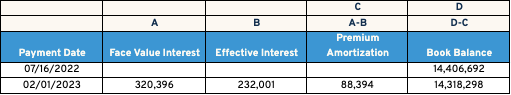Our Face Value Interest of \$320,396 less our Effective Interest of \$232,001 results in a premium amortization of \$88,394 on our first interest payment date on 2/1/2023. We can then reduce our premium balance and book balance by the \$88,394 premium amount, to give us our remaining outstanding Book Balance of \$14,318,298 on 2/1/2023.

This calculation is then continued through the maturity date of the bonds. See the full premium amortization table for the 8/1/2035 maturity below: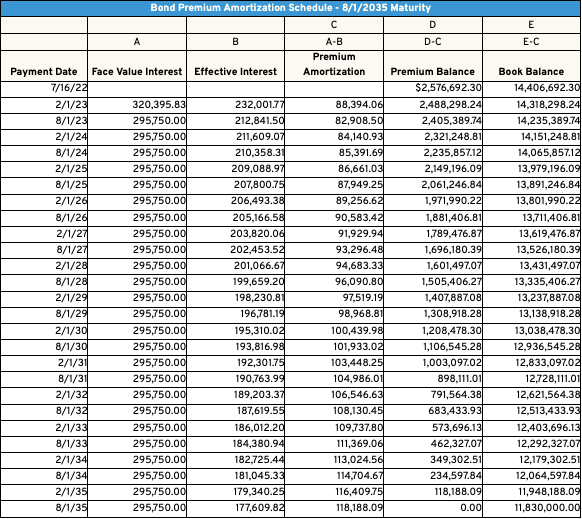Provided below is the same calculation for our 8/1/2041 maturity, which is amortized to maturity as a discount bond.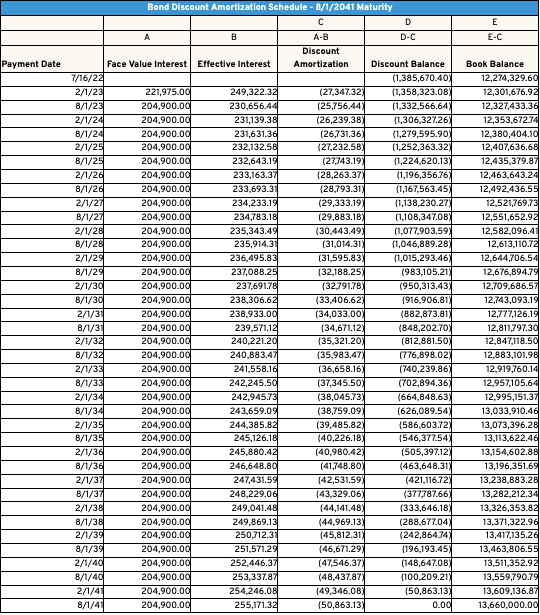These calculations are applied individually to each maturity within a series when using the Effective Interest Rate method. See below for our total premium/discount amortization schedule for our Series 2022 issue.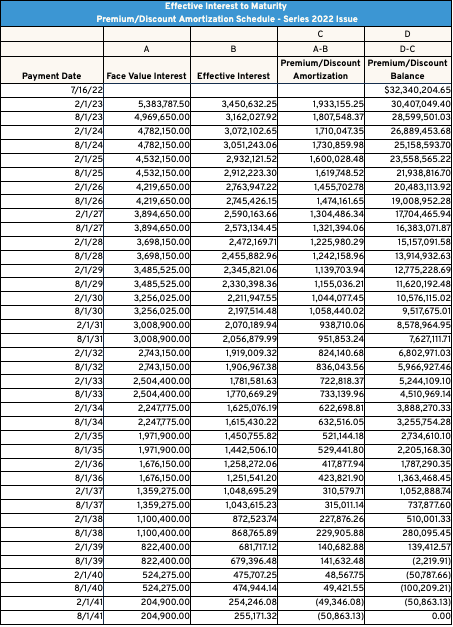## Effective Interest Rate to Call

DebtBook also offers another acceptable form of the Effective Interest method that takes into account the callability of maturities. In the event a bond is a callable premium bond, there is a higher likelihood that the bond will be called before or at the call date. DebtBook’s Effective Interest Rate to Call method amortizes the premium on these maturities with that likelihood in mind.

Callable Bonds. The Effective Interest Rate to Call method takes into account if the stated yield on a maturity  is the Yield to Call or Yield to Maturity. As previously discussed, Municipal bonds are required to be reported at their “yield to worst”, or lowest yield. In the event a premium bond (coupon > yield) is callable, in the overwhelming majority of cases that bond’s stated yield will reflect that it is redeemed at its call date, and not its actual maturity date. This results in a yield that is “worst”, or lower, on the bond's call date, as opposed to the yield if the bond was redeemed at maturity. Let’s take a look again at the pricing of our Series 2022 Water and Sewer Revenue Bond Series which was issued on 7/16/2022: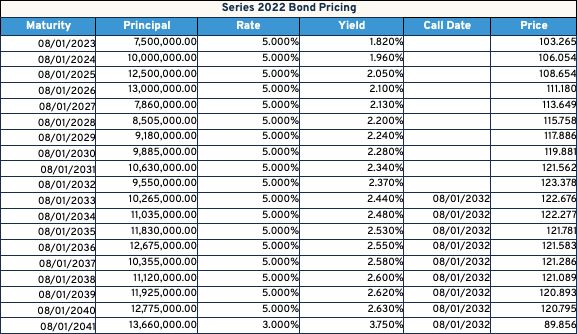The 8/1/2035 maturity of our bond series has a 5.00% coupon and a stated 2.53% yield, and is callable on 8/1/2032. The 2.53% yield represents the yield assuming the bond is called on 8/1/2032, as compared to the Yield to Maturity which assumes the bond is outstanding until maturity. As the yield to call represents the lower yield or “yield to worst”, this is the yield that is used in the Effective Interest to Call Methodology.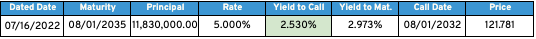The opposite is true for bonds that are callable but structured as discount (coupon<yield) bonds. See the example below. As the “yield to worst” on a discount bond is the yield to maturity and not the yield to call, the price of a callable discount bond is calculated off of the yield to maturity, and thereby the discount is generated.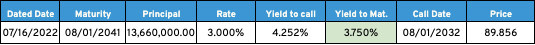So using our example above, our callable premium bond maturing on 08/01/2035 would amortize our premium to 08/01/2032 in full, the call date of the issue. In the case of our discount bond, we would amortize the discount amount to 08/01/2041, the maturity date of the bond. Note non-callable bonds will always be amortized to their maturity date as there is no alternative redemption date available.

In summary, the math outlined in the “Effective Interest Rate to Maturity” method is the same math utilized in Effective Interest Rate to Call, the only change is callable premium bonds will amortize in full at the call date of the maturity, and not the actual maturity date. In the event you do refund these maturities in advance or on the call date, the associated premium will either be fully amortized or nearly fully amortized on that date as opposed to the Effective Interest Rate to Maturity method.

See below for our total premium/discount amortization schedule for our Series 2022 issue using our Effective Interest Rate to Call method. Note the dynamic of the premium amortization rolling off on the call date, and the discount amortization continuing thereafter, reflective of the dynamic we discussed above.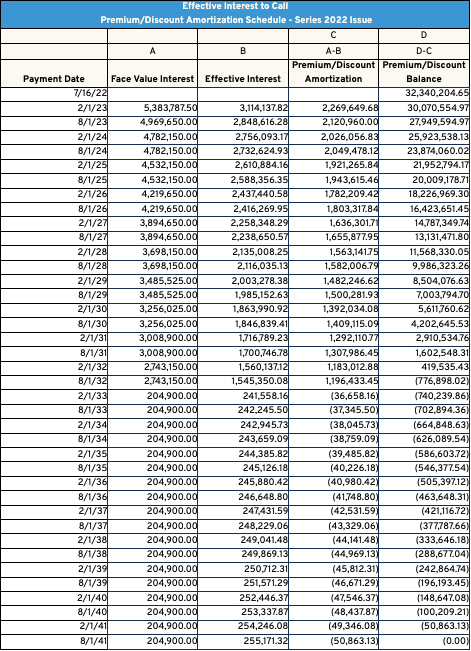## Straight-Line Method

While the Effective Interest Rate method provided in DebtBook is the “correct” approach per the standard, we know in practice many issuers utilize the “Straight-Line” method when calculating their premium/discount amortization.

As is to be expected, the calculation for Straight-Line is more straightforward than the Effective Rate Method outlined above. In the case of Straight-Line, the total net premium/discount is simply divided by the number of days from the dated date, to the final maturity date, thereby generating a level or “Straight-Line” amortization across the life of the bonds. We can use the example of the Series 2022 Bonds we used for our effective interest rate calculations.

Our Series 2022 Bonds have a total net premium amount of \$32,340,205 with a dated date of 7/16/2022, and a final maturity date of 8/1/2041. Assuming a 30/360 day count, there’s a total of 6,855 days from our dated date to our final maturity date. When we divide our total premium of \$32,340,205 by 6,855 days (assuming a 30/360 day count), we will be amortizing \$4,717.75 per day of our net premium. By amortizing daily, we can see our straight-line amortization at any date alignment and frequency we prefer. The graphic below shows the amortization on our payment dates (actual frequency) and on an annual frequency.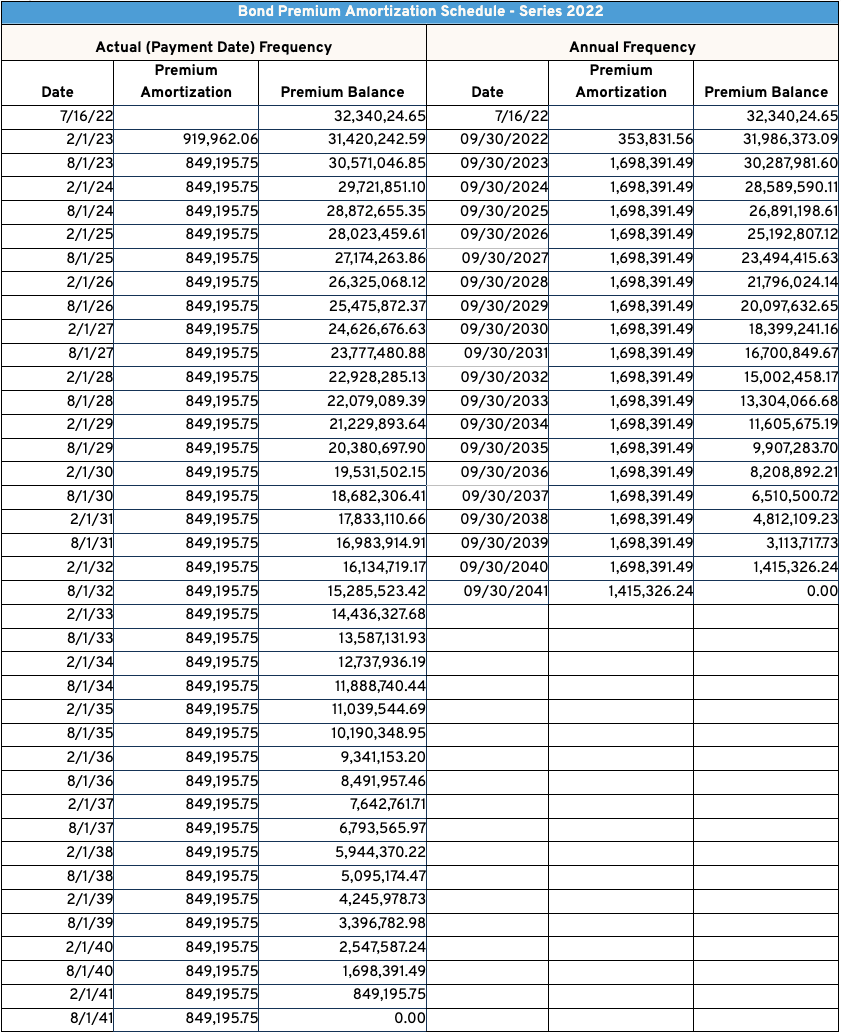## Straight-Line by Maturity Method

In our ongoing effort to constantly improve the application based on client feedback, on April 5, 2023 DebtBook released a new Premium/Discount Amortization method of “Straight-Line by Maturity”.

Straight-Line by Maturity represents a blend of both Effective Interest and Straight-Line methodologies, in that similar to the Effective Interest Rate method outlined above and as implied by the name, Straight-Line by Maturity amortizes the Premium/Discount amount of each maturity to the respective maturity date. In Straight-Line by Maturity, the total net premium/discount for each maturity is simply divided by the number of days from the dated date, to each maturity's respective maturity date, thereby generating a level or “Straight-Line” amortization across the life of that individual maturity. We can use our same example Series 2022 issue to show the calculations.

Let's take a look at the calculation of the amortization on our 8/1/2035 maturity. The 8/1/2035 maturity was issued with a net premium amount of \$2,576,692.30. Assuming a 30/360 day count, there’s a total of 4,695 days from 7/16/2022 (our Dated Date) through 8/1/2035 (our maturity date), resulting in a daily amortization amount of \$548.82. The graphic below shows how the 8/1/2035 is amortized on a “Straight-Line'' on an Actual Payment Frequency: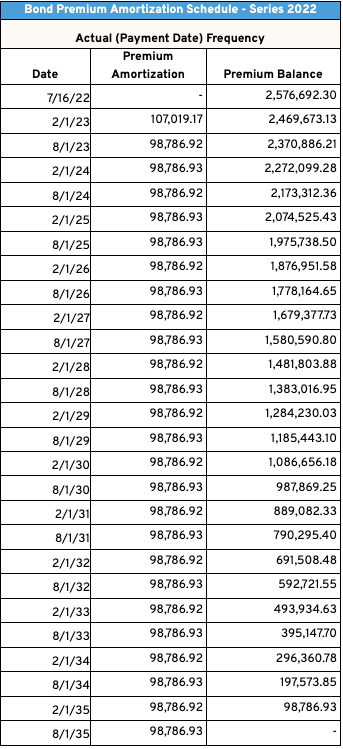These calculations are applied individually to each maturity within a series when using this Straight-Line by Maturity method. See below for our total premium/discount amortization schedule for our Series 2022 issue on both an Actual and Annual Frequency. Note the dynamic of the premium/discount amortization decreasing on each principal payment date when viewing the Actual frequency schedule, reflecting the amortization’s ending for that maturity on its maturity date: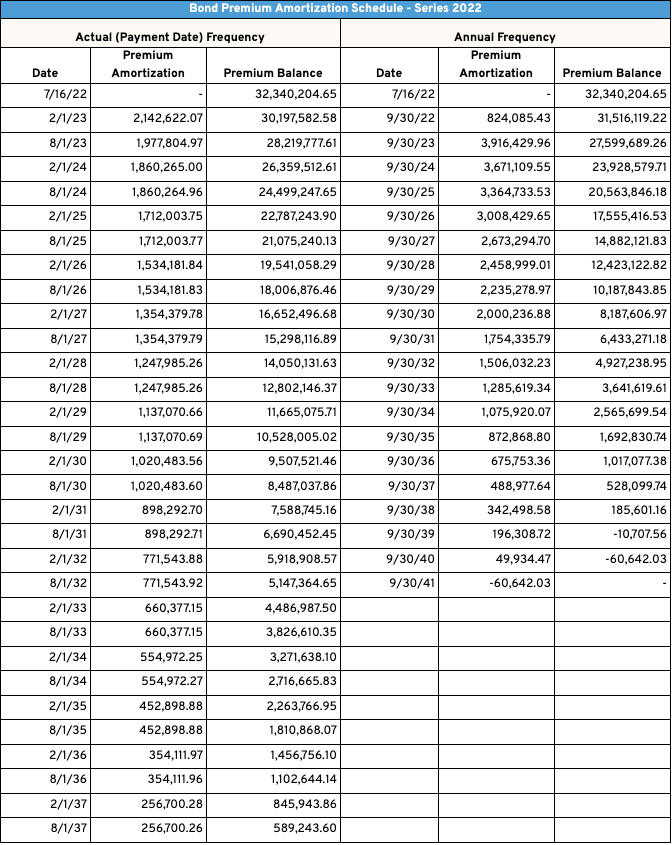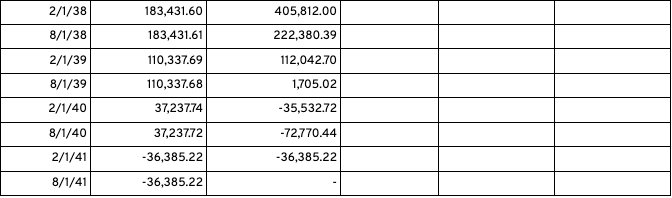Amortization Start Date. When utilizing straight-line methodology, we also give clients the flexibility to set when they want to begin the net premium/discount amortization. The default setting in the application will start amortizing your net premium/discount on the bond’s Dated Date. Again, keeping in mind the application amortizes premium/discount daily, if our bond is dated on 7/16/2022 like in our example, the application would amortize 15 days of premium/discount (assuming a 30/360 day count) in July, then a level 30 days in each month thereafter. As an alternative, DebtBook offers the option of “First of the Month after Dated Date”, which in our scenario above would begin amortizing the bonds on 8/1/2022, and show no amortization in July. As we’re shifting 15 days of amortization, this results in slightly different amortization schedules on a monthly basis depending on which approach you choose.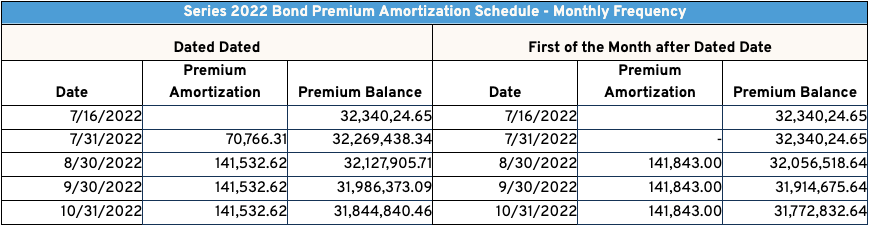Day Count Methodology. As we amortize our premium/discount daily, DebtBook gives you the option of utilizing a 30/360 day count or an Actual/Actual day count when generating your premium/discount amortization schedules when using either Effective Interest or Straight-Line methodologies. The graphic below demonstrates the difference between both methods when viewing the Straight-Line amortization on a monthly basis for the first 4 months the issue is outstanding. As you would expect, utilizing “Actual/Actual” day count amortizes premium based on the actual days of the month (31 days of amortization in October), vs. 30/360 which assumes a level 30 days in every month (30 days of amortization in October).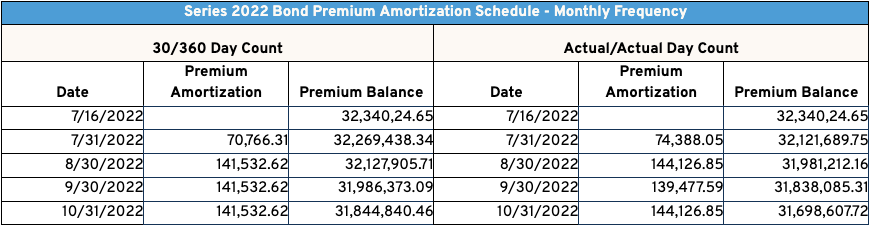Disclaimer: DebtBook does not provide professional services or advice. DebtBook has prepared these materials for general informational and educational purposes, which means we have not tailored the information to your specific circumstances. Please consult your professional advisors before taking action based on any information in these materials. Any use of this information is solely at your own risk.